R script: reset.R

$$\newcommand{\esp}{\mathbb{E}\left(#1\right)} \newcommand{\var}{\mbox{Var}\left(#1\right)} \newcommand{\deriv}{\dot{#1}(t)} \newcommand{\prob}{ \mathbb{P}\!(#1)} \newcommand{\eqdef}{\mathop{=}\limits^{\mathrm{def}}} \newcommand{\by}{\boldsymbol{y}} \newcommand{\bc}{\boldsymbol{c}} \newcommand{\bpsi}{\boldsymbol{\psi}} \def\pmacro{\texttt{p}} \def\like{{\cal L}} \def\llike{{\cal LL}} \def\logit{{\rm logit}} \def\probit{{\rm probit}} \def\one{{\rm 1\!I}} \def\iid{\mathop{\sim}_{\rm i.i.d.}} \def\simh0{\mathop{\sim}_{H_0}} \def\df{\texttt{df}} \def\res{e} \def\xomega{x} \newcommand{\argmin}{{\rm arg}\min_{#1}} \newcommand{\argmax}{{\rm arg}\max_{#1}} \newcommand{\Rset}{\mbox{\mathbb{R}}} \def\param{\theta} \def\setparam{\Theta} \def\xnew{x_{\rm new}} \def\fnew{f_{\rm new}} \def\ynew{y_{\rm new}} \def\nnew{n_{\rm new}} \def\enew{e_{\rm new}} \def\Xnew{X_{\rm new}} \def\hfnew{\widehat{\fnew}} \def\degree{m} \def\nbeta{d} \newcommand{\limite}{\mathop{\longrightarrow}\limits_{#1}} \def\ka{k{a}} \def\ska{k{\scriptscriptstyle a}} \def\kel{k{e}} \def\skel{k{\scriptscriptstyle e}} \def\cl{C{\small l}} \def\Tlag{T\hspace{-0.1em}{lag}} \def\sTlag{T\hspace{-0.07em}{\scriptscriptstyle lag}} \def\Tk{T\hspace{-0.1em}{k0}} \def\sTk{T\hspace{-0.07em}{\scriptscriptstyle k0}} \def\thalf{t{1/2}} \newcommand{\Dphi}{\partial_\pphi #1} \def\asigma{a} \def\pphi{\psi} \newcommand{\stheta}{{\theta^\star}} \newcommand{\htheta}{{\widehat{\theta}}}$$

# 1 Introduction

Macros depot and empty can be used for respectively fill and empty compartments defined as components of an ODE system. On the other hand, reset is used for resetting components of the system to their initial values.

These macros can be combined in a same model.

# 2 Filling compartments

## 2.1 Filling a single compartment

Consider a one compartment model for oral administration:

pk.model1 <- inlineModel("
[LONGITUDINAL]
input = {ka, k}

PK:

EQUATION:
")

Here, we are interested in the amount in the central compartment

p <- c(ka=0.3,  k=0.1)
out <- list(name= "Ac", time = seq(0,150,0.1))

By default, the target is Ad, which means that all the doses go to the depot compartment

trt <- list(amt=100, time=seq(10,136,by=24))

Let us compute and plot the amount in the central compartment defined by this model and this dosing regimen:

r <- simulx(model=pk.model1, parameter = p, treatment = trt, output = out)
ggplot(r$Ac, aes(time,Ac)) + geom_line()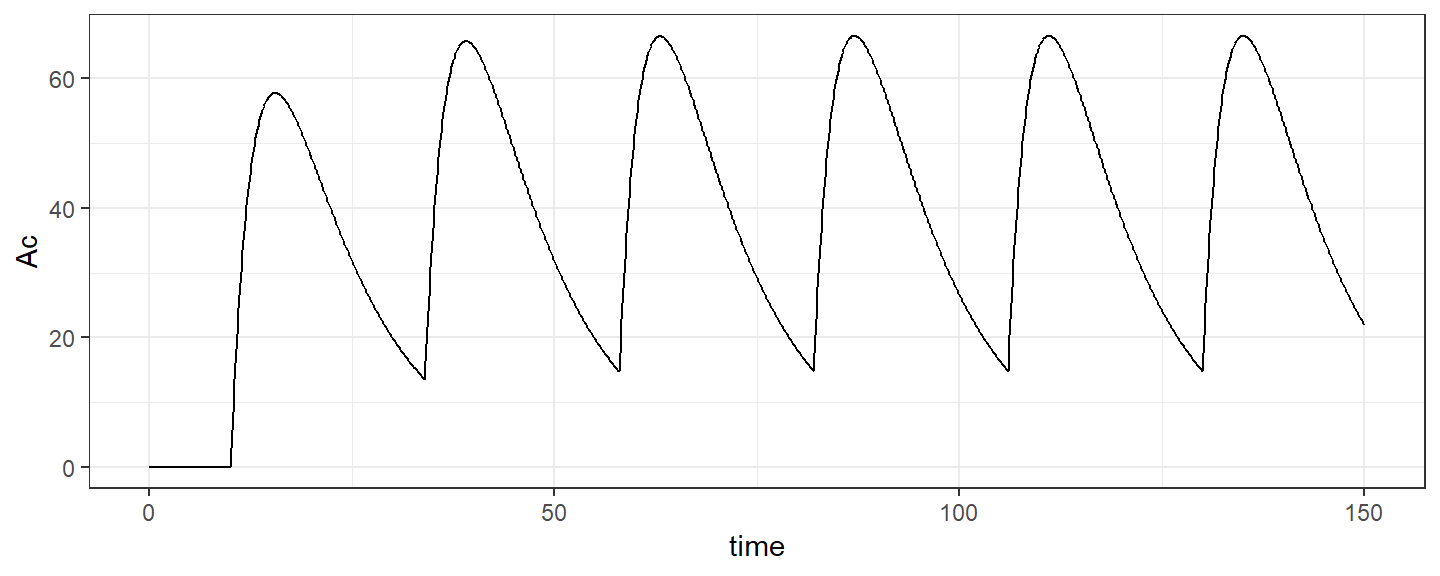## 2.2 Filling multiple compartments We can now consider both oral (target=Ad) and iv (target=Ac) administrations for this PK model: pk.model2 <- inlineModel(" [LONGITUDINAL] input = {ka, k} PK: depot(target=Ad, type=1) depot(target=Ac, type=2) EQUATION: ddt_Ad = -ka*Ad ddt_Ac = ka*Ad - k*Ac ") We can then define and combine oral (type=1) and iv (type=2) of administrations: trt1 <- list(amt=100, time=seq(10,136,by=24), type=1) # put doses in the depot compartment trt2 <- list(amt=50, time=seq(28,136,by=48), type=2) # put doses in the central compartment trt <- list(trt1, trt2) r <- simulx(model=pk.model2, parameter = p, treatment = trt, output = out) ggplot(r$Ac, aes(time,Ac)) + geom_line()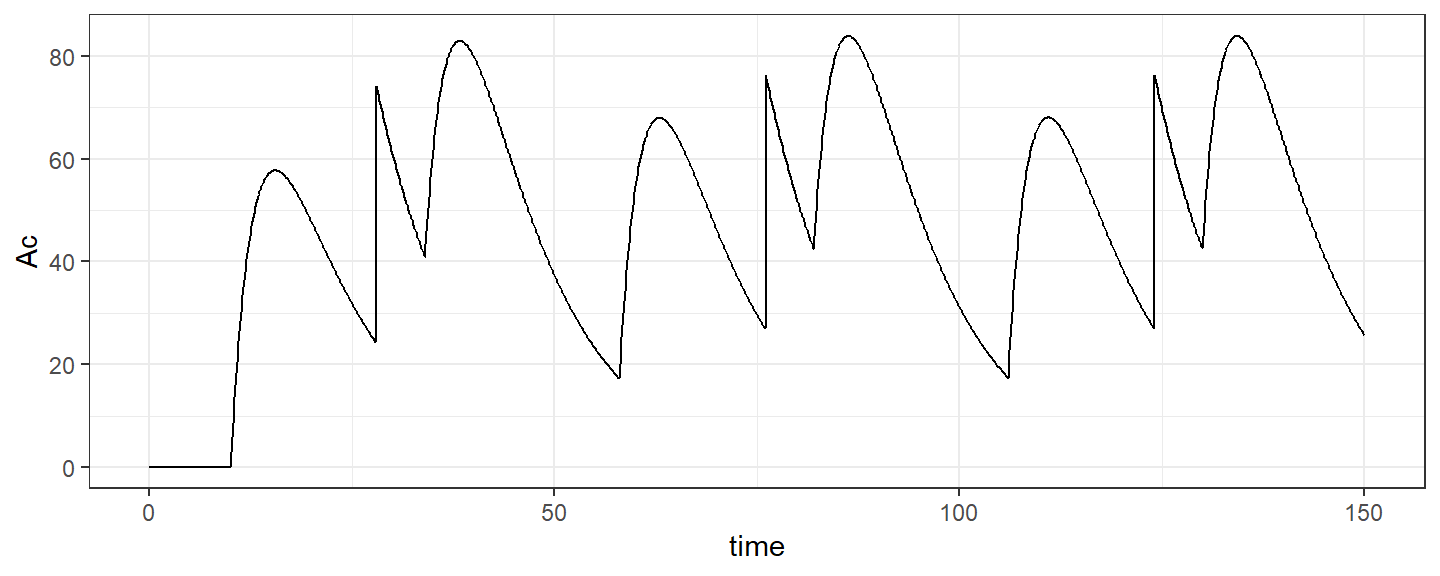# 3 Emptying compartments

## 3.1 Emptying a single compartment

Any component of the system can be set to 0 at any time. In this example, Ac can be set to 0, which means that the central compartment cen be empty:

pk.model1 <- inlineModel("
[LONGITUDINAL]
input = {ka, k}

PK:
empty(target=Ac, type=2)

EQUATION:
")

p <- c(ka=0.3,  k=0.1)
out <- list(name= "Ac", time = seq(0,150,0.1))

Here, trt1 defines the doses which are administered

trt1 <- list(amt=100, time=seq(10,136,by=24), type=1)

while trt2 defines the time at which the central compartment is emptied

trt2 <- list(amt=0, time=seq(28,136,by=48), type=2)

Both actions can then be combined:

trt <- list(trt1, trt2)
r <- simulx(model=pk.model1, parameter = p, treatment = trt, output = out)
ggplot(r$Ac, aes(time,Ac)) + geom_line()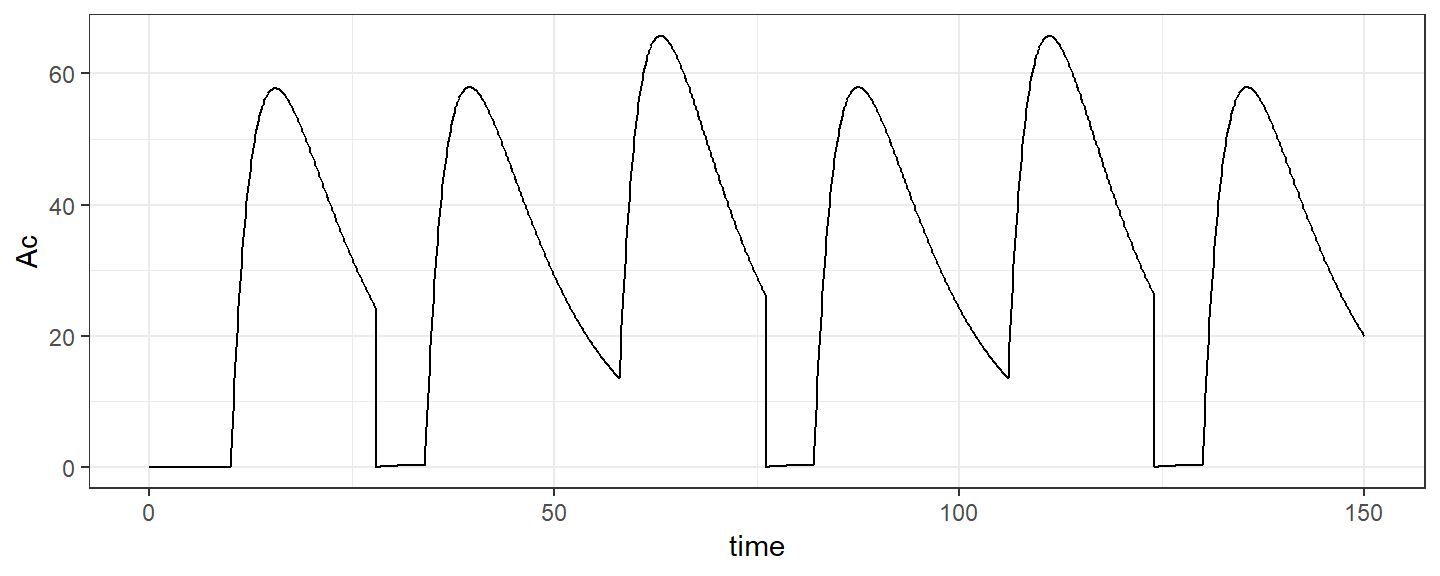Remark: With mlxR <= 3.3.3, it is mandatory to define the amount for trt2 even if it is ignored (use amt=0 for instance). It is not necessary with mlxR >= 3.3.4: # mlxR >= 3.3.4 allows the following definition of emptying actions: trt2 <- list(time=seq(28,136,by=48), type=2) ## 3.2 Emptying multiple compartments It is possible to set several, or even all, components of the system to 0 pk.model2 <- inlineModel(" [LONGITUDINAL] input = {ka, k} PK: depot(target=Ad, type=1) empty(target=Ad, type=2) empty(target=Ac, type=3) empty(target=all, type=4) EQUATION: ddt_Ad = -ka*Ad ddt_Ac = ka*Ad - k*Ac ") p <- c(ka=0.3, k=0.1) out <- list(name= c("Ad", "Ac"), time = seq(0,150,0.1)) Here, we define several sequences of actions: trt1 <- list(amt=100, time=seq(10,136,by=24), type=1) # put doses in the depot compartment trt2 <- list(amt=0, time=seq(38,136,by=48), type=2) # empty the depot compartment trt3 <- list(amt=0, time=seq(38,136,by=48), type=3) # empty the central compartment trt4 <- list(amt=0, time=seq(38,136,by=48), type=4) # empty all the compartments In this first example, only the depot compartment is emptied at various times: library(gridExtra) trt <- list(trt1, trt2) r <- simulx(model=pk.model2, parameter = p, treatment = trt, output = out) pl1 <- ggplot(r$Ad, aes(time,Ad)) + geom_line()
pl2 <- ggplot(r$Ac, aes(time,Ac)) + geom_line() grid.arrange(pl1, pl2)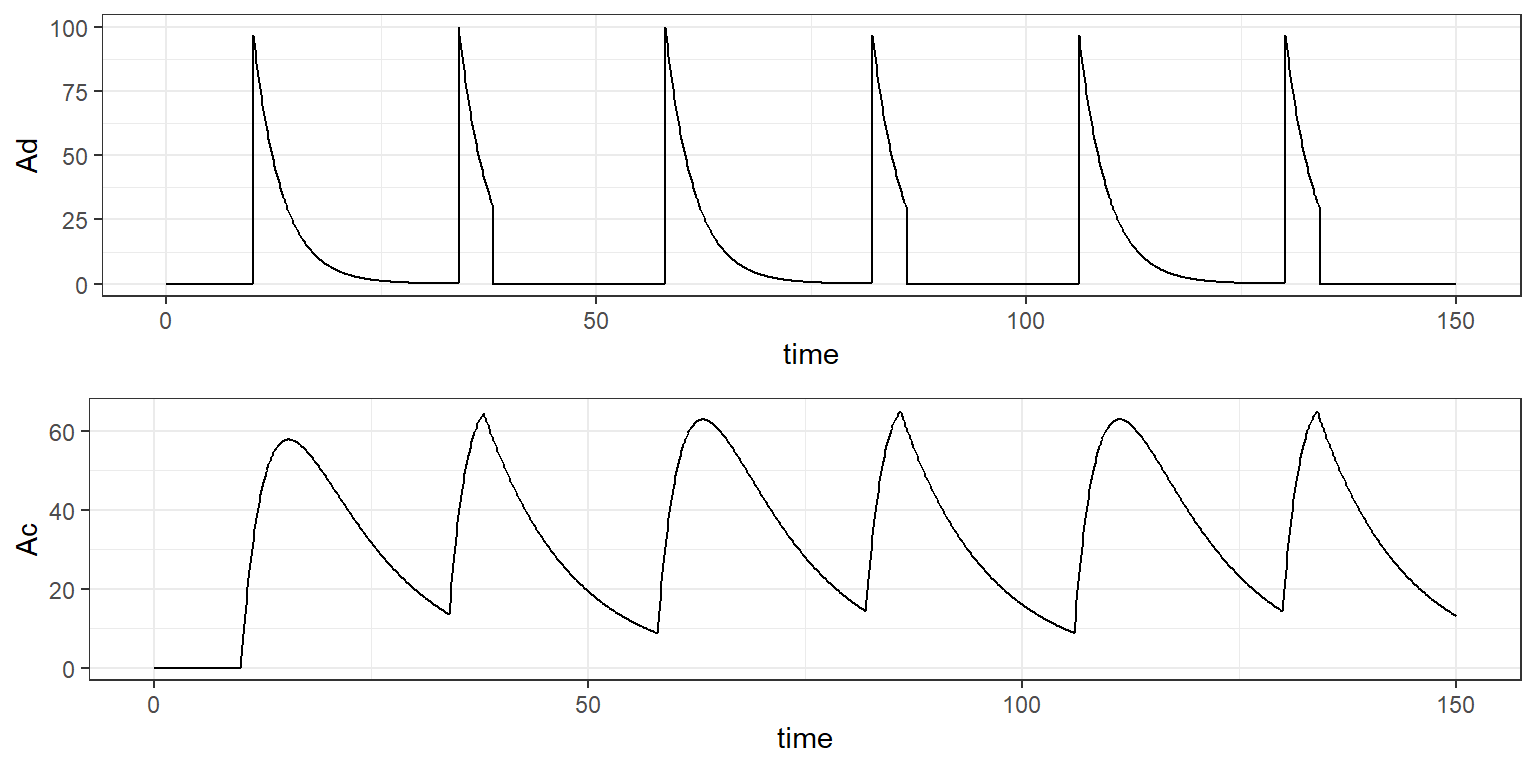In this second example, only the central compartment is emptied at various times: trt <- list(trt1, trt3) r <- simulx(model=pk.model2, parameter = p, treatment = trt, output = out) pl1 <- ggplot(r$Ad, aes(time,Ad)) + geom_line()
pl2 <- ggplot(r$Ac, aes(time,Ac)) + geom_line() grid.arrange(pl1, pl2)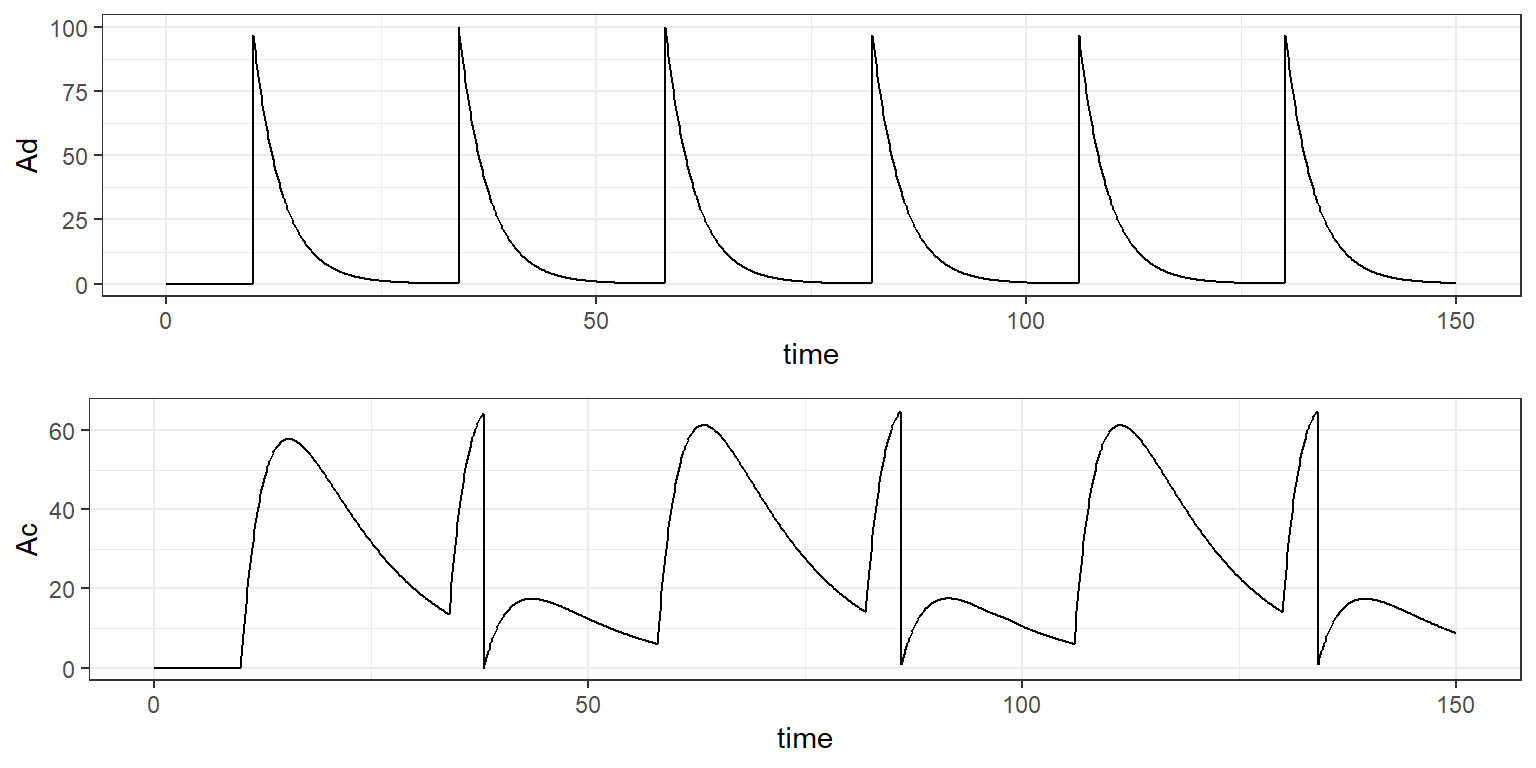In this last example, all the compartments are emptied simultaneously at various times: trt <- list(trt1, trt4) r <- simulx(model=pk.model2, parameter = p, treatment = trt, output = out) pl1 <- ggplot(r$Ad, aes(time,Ad)) + geom_line()
pl2 <- ggplot(r$Ac, aes(time,Ac)) + geom_line() grid.arrange(pl1, pl2)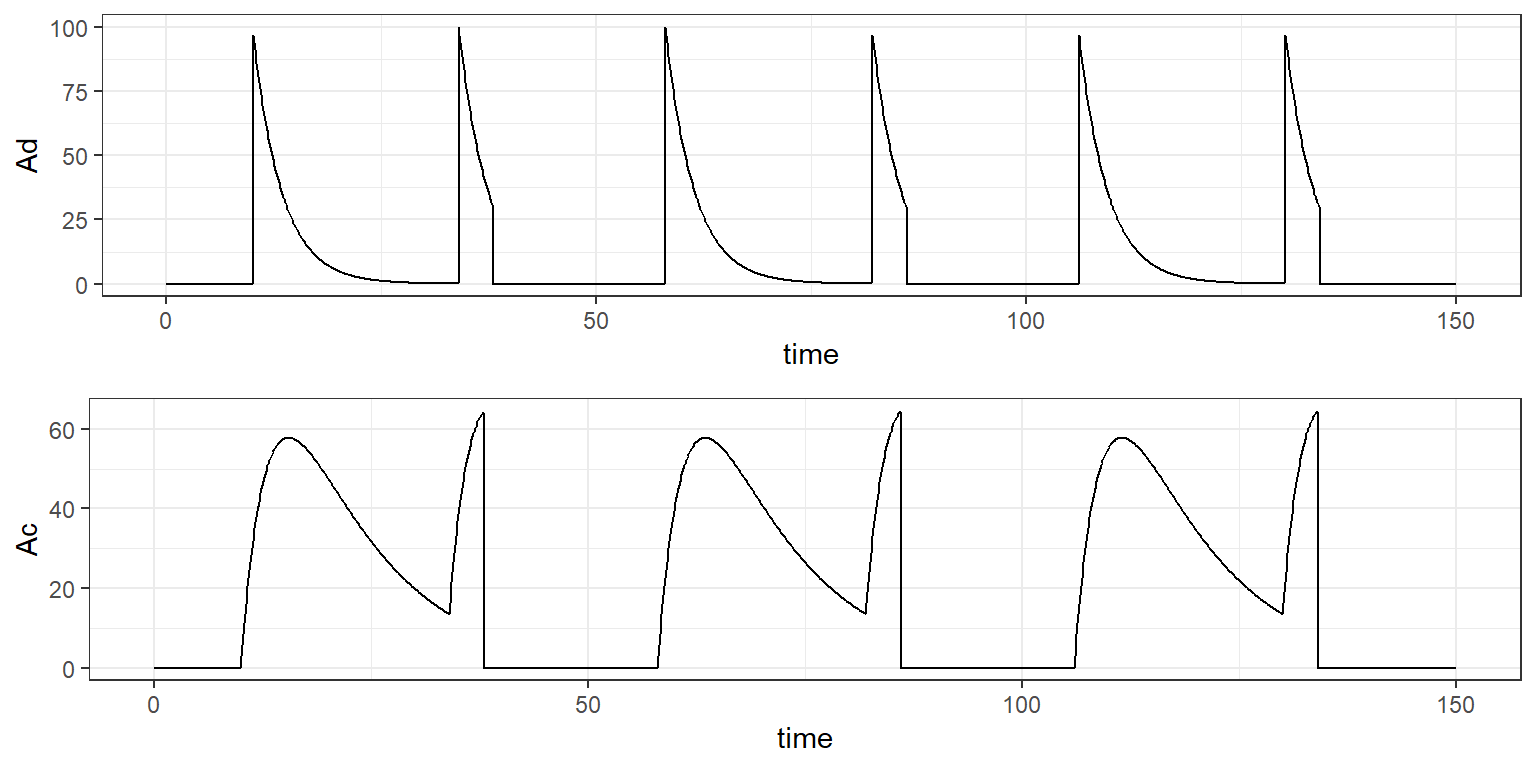# 4 Resetting compartments ## 4.1 Resetting a single compartment Instead of setting a component to 0, it is possible to reset it to its nitial values using the reset macro. Of course, emptying a compartment of resetting it to its initial state are equivalent actions for compartment which are initially empty. This may be not the case for PD models with non-zero baseline pkpd.model1 <- inlineModel(" [LONGITUDINAL] input = {ka, k, E0, IC50, kout} PK: depot(target=Ad, type=1) reset(target=E, type=2) EQUATION: E_0 = E0 kin = E0*kout ddt_Ad = -ka*Ad ddt_Ac = ka*Ad - k*Ac ddt_E = kin*(1 - Ac/(Ac+IC50)) - kout*E ") p <- c(ka=0.3, k=0.1, E0=100, IC50=20, kout=0.2) out <- list(name="E", time = seq(0,150,0.1)) Let us define the dosing regimen trt1 <- list(amt=100, time=seq(10,136,by=24), type=1) and compute the predicted effect r <- simulx(model=pkpd.model1, parameter = p, treatment = trt1, output = out) ggplot(r$E, aes(time,E)) + geom_line()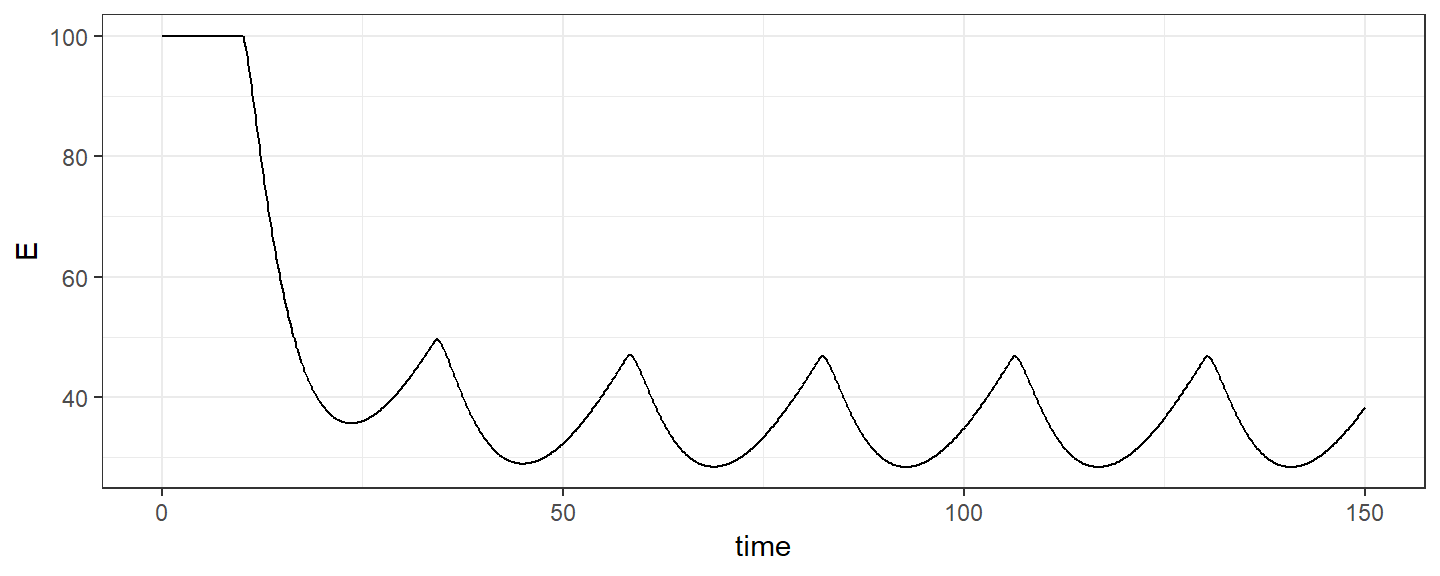Imagine now that component $$E$$ is reset to its initial value $$E_0$$ at various times:

trt2 <- list(amt=0,   time=seq(60,136,by=48), type=2)

We can then combine these two types of action:

trt <- list(trt1, trt2)
r <- simulx(model=pkpd.model1, parameter = p, treatment = trt, output = out)
ggplot(r$E, aes(time,E)) + geom_line()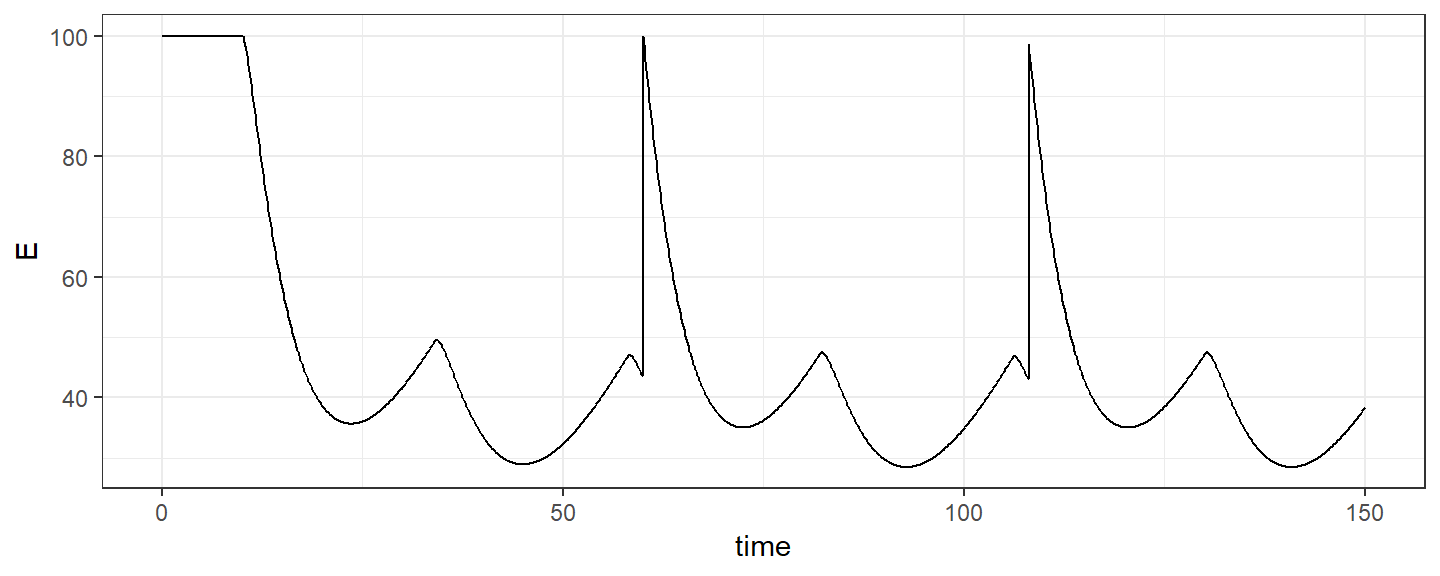## 4.2 Resetting multiple compartments Several, or all, components of the system can be reset to their initial values: pkpd.model2 <- inlineModel(" [LONGITUDINAL] input = {ka, k, E0, IC50, kout} PK: depot(target=Ad, type=1) reset(target=E, type=2) reset(target=Ac, type=3) reset(target=all, type=4) EQUATION: E_0 = E0 kin = E0*kout ddt_Ad = -ka*Ad ddt_Ac = ka*Ad - k*Ac ddt_E = kin*(1 - Ac/(Ac+IC50)) - kout*E ") p <- c(ka=0.3, k=0.1, E0=100, IC50=20, kout=0.2) out <- list(name=c("Ad", "Ac", "E"), time = seq(0,150,0.1)) We can define several sequences of actions trt1 <- list(amt=100, time=seq(10,136,by=24), type=1) trt2 <- list(amt=0, time=seq(60,136,by=48), type=2) trt3 <- list(amt=0, time=seq(60,136,by=48), type=3) trt4 <- list(amt=0, time=seq(60,136,by=48), type=4) and combine them, by resetting, for instance, $$A_c$$ and $$E$$ to their initial values. trt <- list(trt1, trt2, trt3) r <- simulx(model=pkpd.model2, parameter = p, treatment = trt, output = out) pl1 <- ggplot(r$Ad, aes(time,Ad)) + geom_line()
pl2 <- ggplot(r$Ac, aes(time,Ac)) + geom_line() pl3 <- ggplot(r$E, aes(time,E)) + geom_line()
grid.arrange(pl1, pl2, pl3)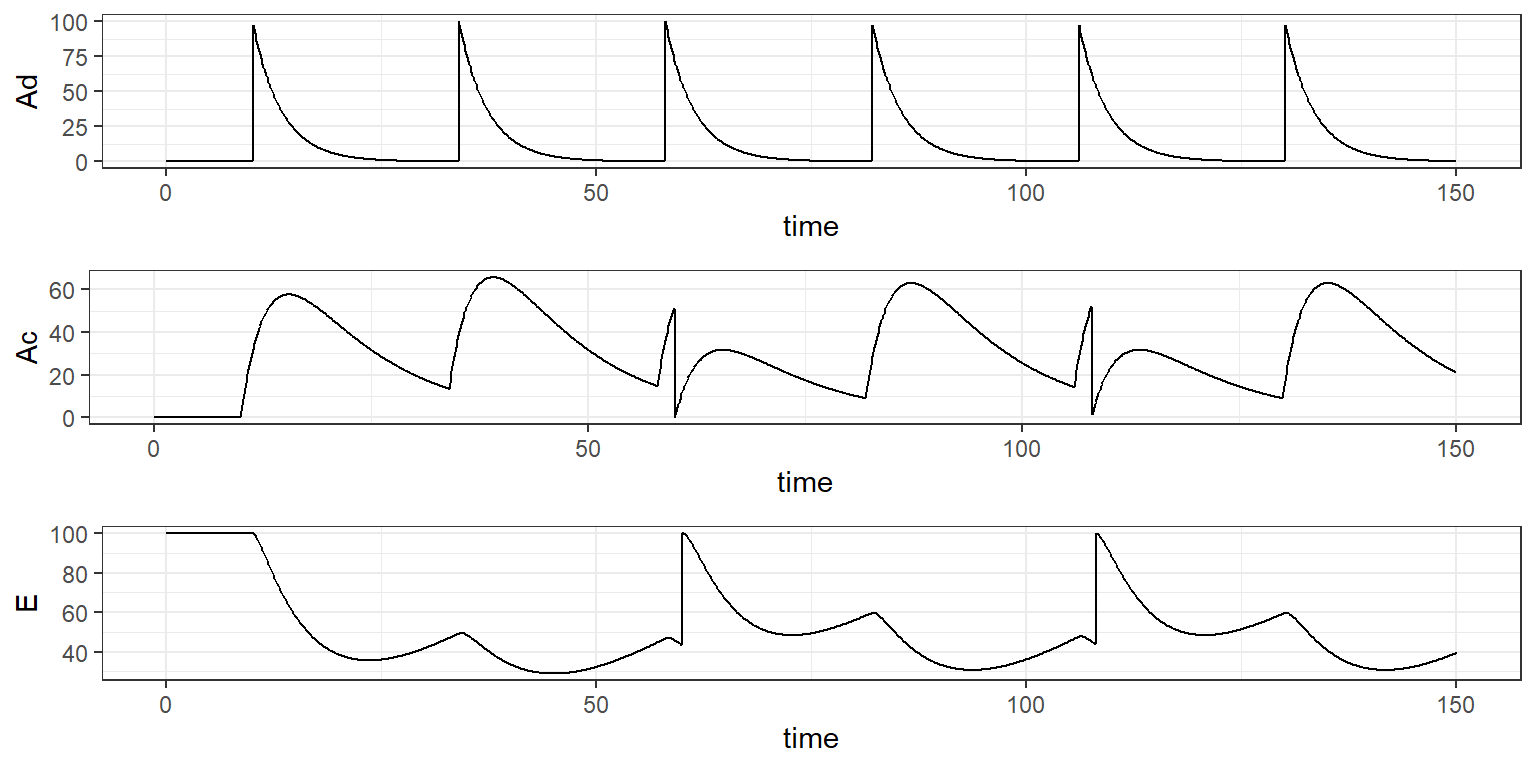Here, all the components of the system are reset to their initial values:

trt <- list(trt1, trt4)
r <- simulx(model=pkpd.model2, parameter = p, treatment = trt, output = out)
pl1 <- ggplot(r$Ad, aes(time,Ad)) + geom_line() pl2 <- ggplot(r$Ac, aes(time,Ac)) + geom_line()
pl3 <- ggplot(r$E, aes(time,E)) + geom_line() grid.arrange(pl1, pl2, pl3)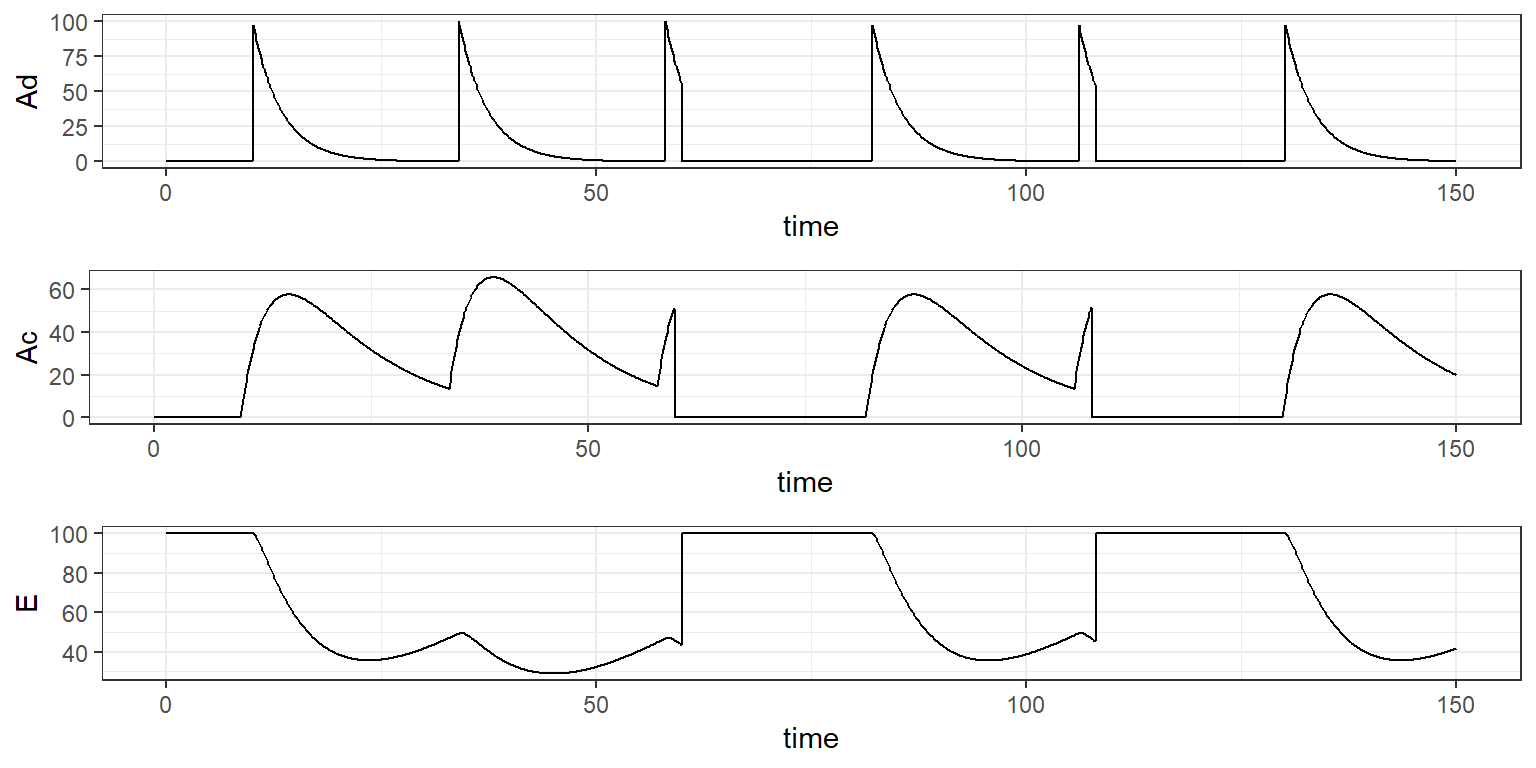Remark: resetting and emptying the depot and central compartments are equivalent actions. Then, we could equivalently define the following actions: pkpd.model2b <- inlineModel(" [LONGITUDINAL] input = {ka, k, E0, IC50, kout} PK: depot(target=Ad, type=1) reset(target=E, type=2) empty(target=Ac, type=3) empty(target=Ad, type=4) empty(target=Ac, type=4) reset(target=E, type=4) EQUATION: E_0 = E0 kin = E0*kout ddt_Ad = -ka*Ad ddt_Ac = ka*Ad - k*Ac ddt_E = kin*(1 - Ac/(Ac+IC50)) - kout*E ") r <- simulx(model=pkpd.model2, parameter = p, treatment = trt, output = out) pl1 <- ggplot(r$Ad, aes(time,Ad)) + geom_line()
pl2 <- ggplot(r$Ac, aes(time,Ac)) + geom_line() pl3 <- ggplot(r$E, aes(time,E)) + geom_line()
grid.arrange(pl1, pl2, pl3)